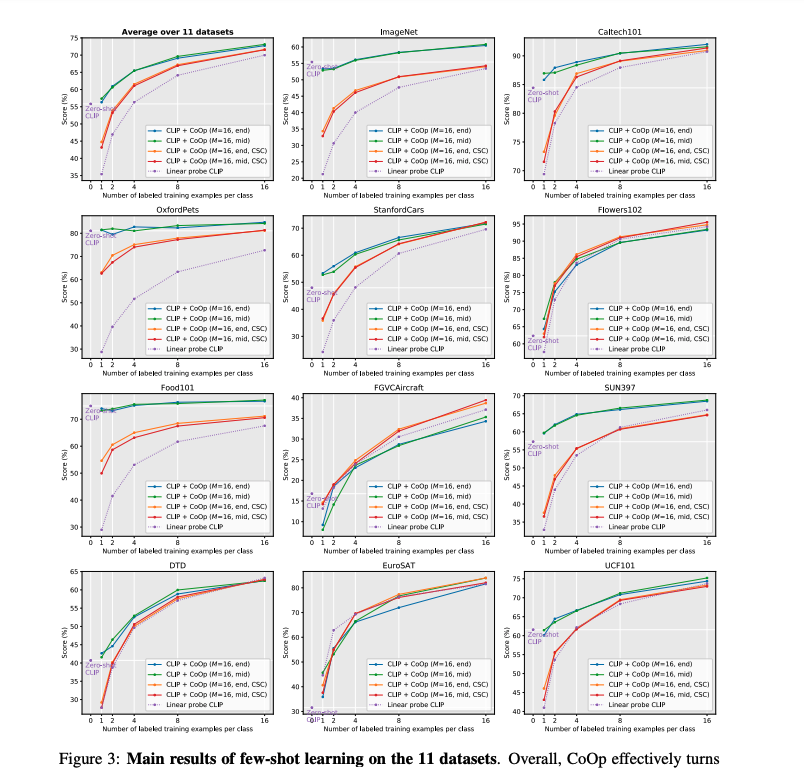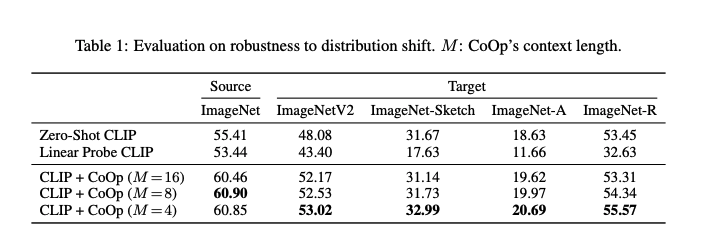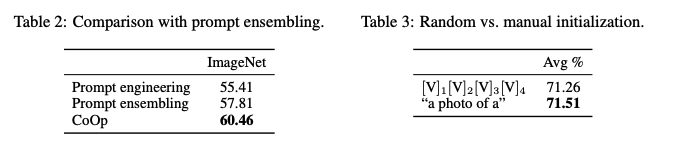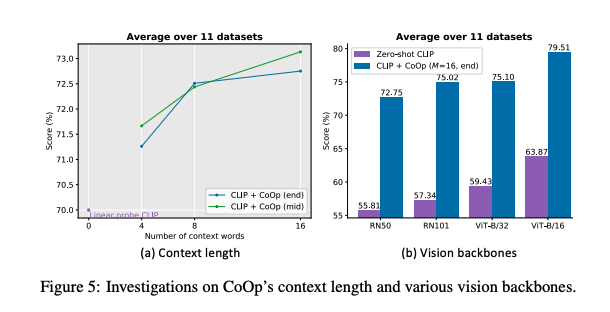## 方法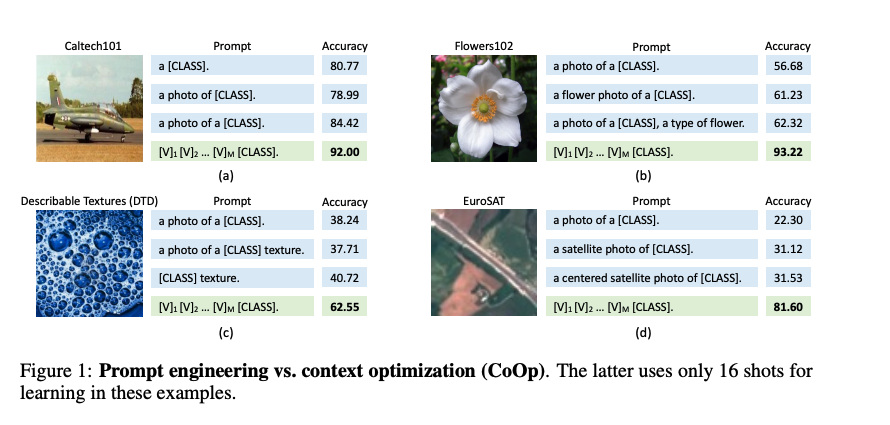1. 从上图a中，对于Caltech101数据的第2个和第3个prompt，在class token之前添加一个 “a” token，准确率提高了超过5%。
2. 从上图b-d，可以看出添加任务相关的上下文可以带来性能的显著提升，如上图b的“flower”、上图c的“texture”和上图d的“satellite”。
3. 从上图b中，在prompt的后面加上 “a type of flower”就能提升性能，说明调整句子结构可以带来进一步的性能改进。
4. 上图c只保留“texture”就能提升性能。
5. 上图d加上一个单词“centered”就能提升性能。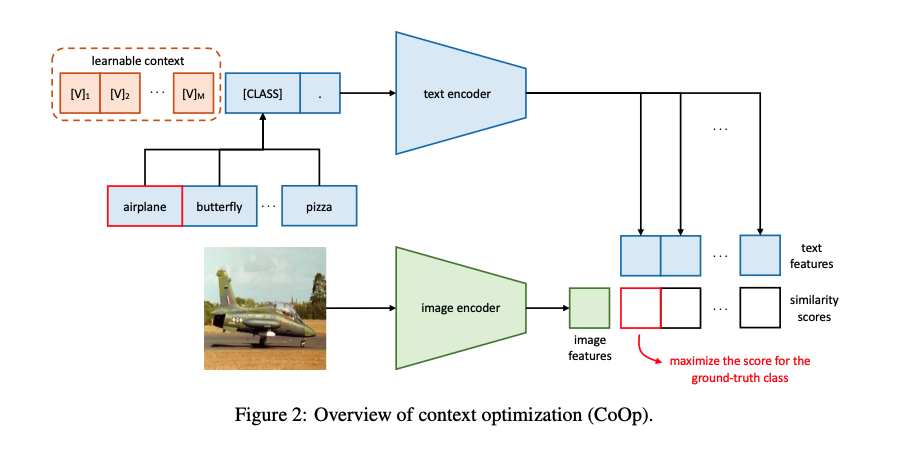$\boldsymbol{t}=[\mathrm{V}]_{1}[\mathrm{~V}]_{2} \ldots[\mathrm{V}]_{M}[\mathrm{CLASS}]$

$p(y=i \mid \boldsymbol{x})=\frac{\exp \left(/ \tau\right)}{\sum_{j=1}^{K} \exp \left(/ \tau\right)}$

$\boldsymbol{t}=[\mathrm{V}]_{1} \ldots[\mathrm{V}]_{\frac{M}{2}}[\mathrm{CLASS}][\mathrm{V}]_{\frac{M}{2}+1} \ldots[\mathrm{V}]_{M}$

$[\mathrm{V}]_{1}^{i}[\mathrm{~V}]_{2}^{i} \ldots[\mathbf{V}]_{M}^{i} \neq[\mathrm{V}]_{1}^{j}[\mathrm{~V}]_{2}^{j} \ldots[\mathrm{V}]_{M}^{j} \text { for } i \neq j \text { and } i, j \in\{1, \ldots, K\}$

## 实验

### 训练细节

1. 将class token放在prompt序列末尾。
2. 将class token放在prompt序列中间。
3. 使用统一的prompt，即参数在所有类别之间共享。
4. 特定类别不同prompt，即CSC。

### 实验结果